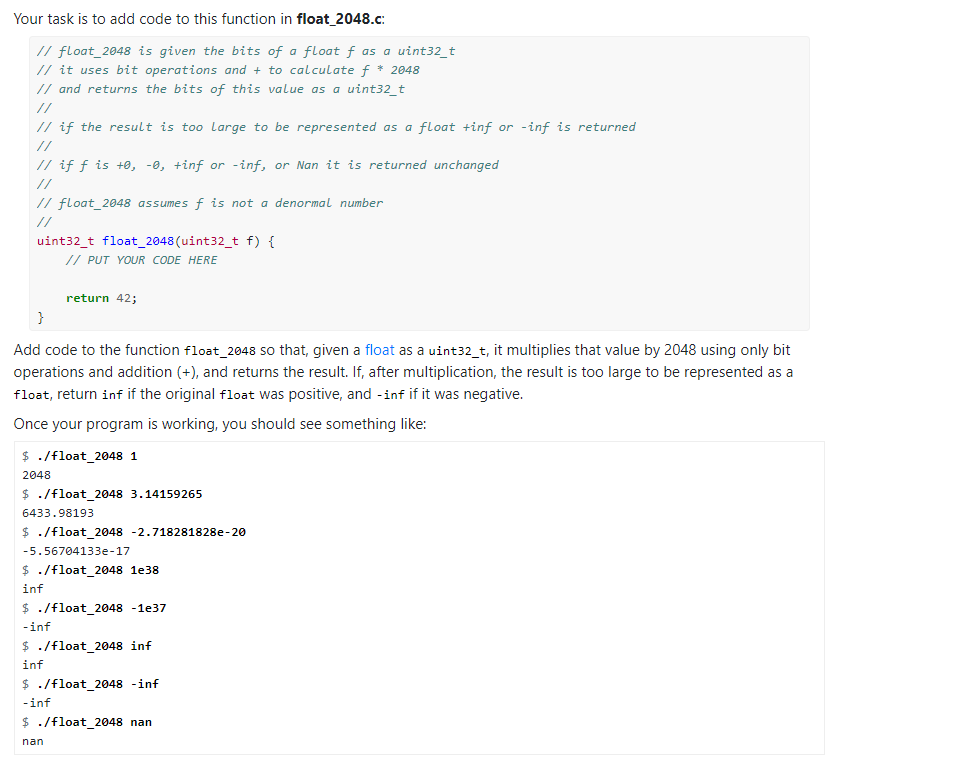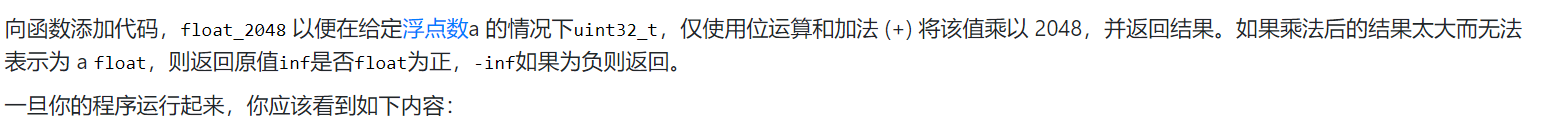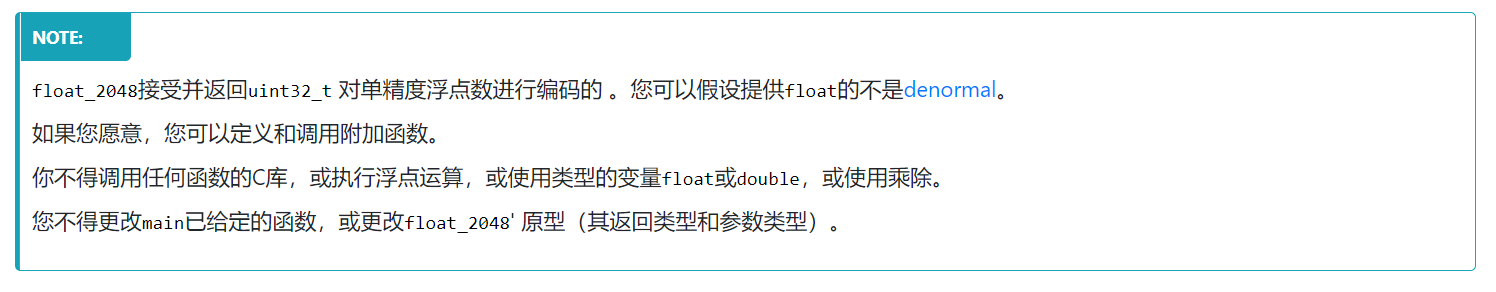###### 啦啦啦拉拉裤
2021-10-03 19:42浏览 46

# C语言问题 使用位运算将浮点数乘以 2048``````
// Multiply a float by 2048 using bit operations only
#include <stdio.h>
#include <stdint.h>
#include <stdlib.h>
#include <assert.h>
#include "floats.h"
// float_2048 is given the bits of a float f as a uint32_t
// it uses bit operations and + to calculate f * 2048
// and returns the bits of this value as a uint32_t
//
// if the result is too large to be represented as a float +inf or -inf is returned
//
// if f is +0, -0, +inf or -inf, or Nan it is returned unchanged
//
// float_2048 assumes f is not a denormal number
//
uint32_t float_2048(uint32_t f) {